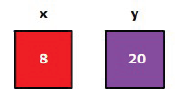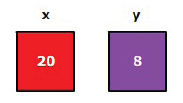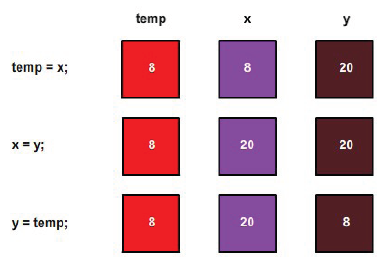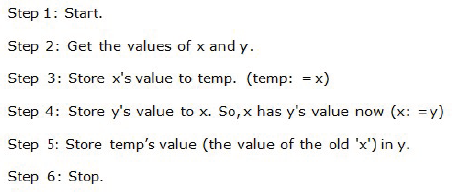#### Exchanging values of two variables

Problem definition: Exchanging values of two variables.

Analysis: Two variables x and y contains two different values.

Swap the values of x and y such that x has y′s value and y has x′s value.

Solving by example: Let us consider two variables x and y,containing values 8 and 20 respectively.

The original values of x and y are:The requirement is once the algorithm is performed, the results should beIf you think by just saying,

x=y; y=x;

The value gets swapped, then you are mistaken.

These instruction are atomic in nature and hence x = y means that the value of ‘x’ is lost.

So,we have to use a temporary variable,temp to store the value of ‘x’.The value of ‘x’ and ‘y’ is swapped.

Algorithm Definition